# Getting Started With Tensorflow – A Machine Learning Library

Machine learning is a complex discipline. But implementing the machine learning models is far less daunting and difficult than it used to be, thanks to the machine learning frameworks—such as Google’s TensorFlow: that ease the process of acquiring data, training models, serving predictions, and future results.

## Tensorflow Basics

TensorFlow is an open-source library for numerical computation and large-scale machine learning. TensorFlow bundles the slew of machine learning and deep learning (also known as neural networking) models and algorithms and makes them useful through the common metaphor.

It uses Python to provide a convenient front-end API for building applications with the framework while executing those applications in high-performance C++.

For this example, I am using Jupyter Notebook to perform some Tensorflow practicals.

So, if your notebook has not installed the Tensorflow library, you can install it using Anaconda Navigator and find the environment section of Anaconda Navigator. You will see something like this.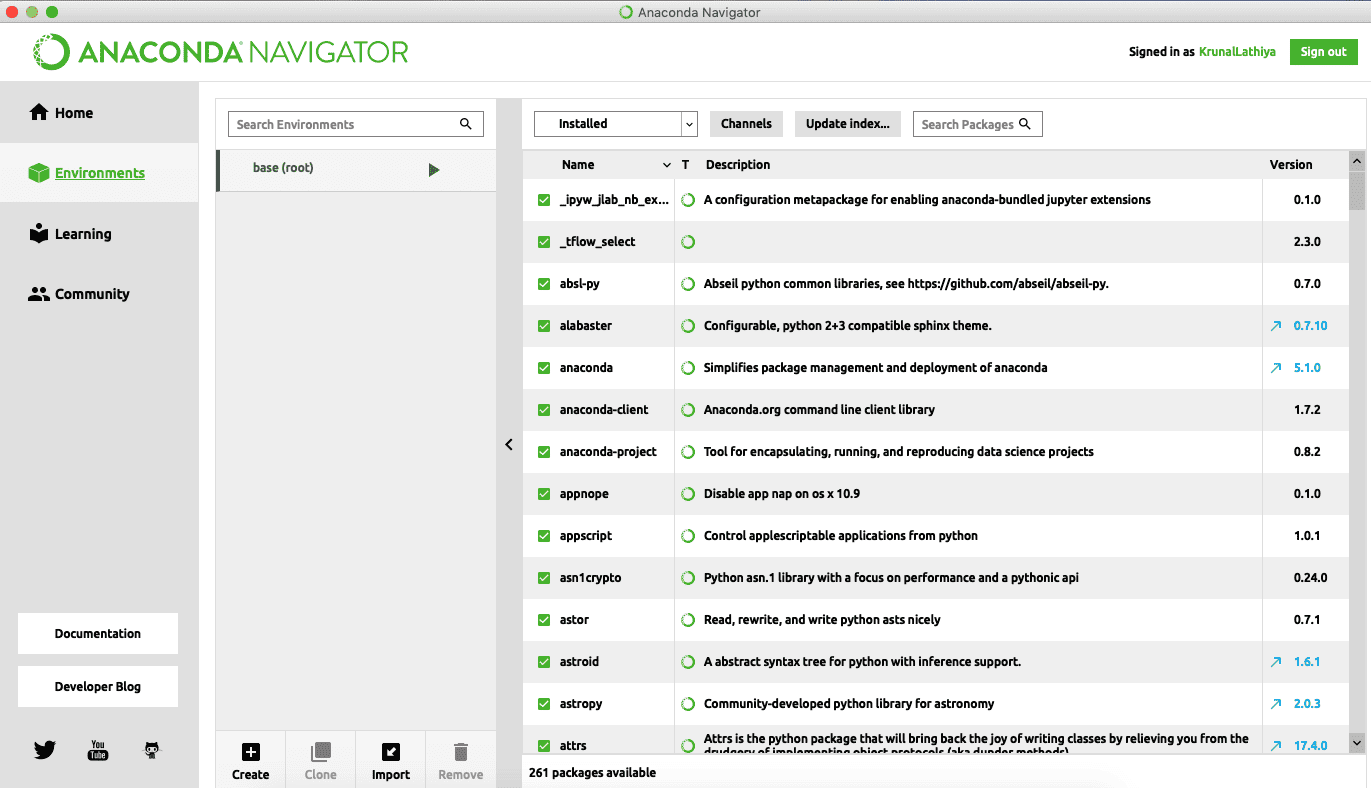Here, if a tensorflow package is uninstalled on your machine, then you can install it from here.

Now, launch a Jupyter Notebook, create a new book, and verify that you have installed the tensorflow using the following code in the first cell of Jupyter Notebook.

```import tensorflow as tf
tf.__version__```

Run the cell using the Ctrl + Enter command and see the output.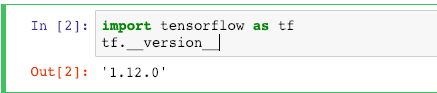## Tensorflow Basic Syntax

Let’s define the Tensorflow constants using the following code. You need to write that code in the next cell.

```app = tf.constant('app')
type(app)```

Here, I have defined the app tensorflow constant, and also we have checked the type of that constant.

Run the cell.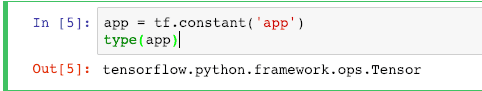Let’s define one more tensorflow constant and see its datatype as well.

```dividend = tf.constant('dividend')
type(dividend)```

See the output below.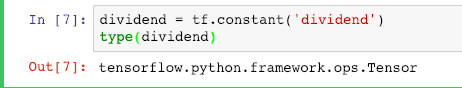Now, print the constant and see the output.

`print(app)`

See the output below.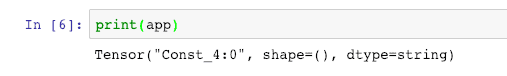See the data type of the constant is String. The object is a Tensor.

## The tf.session in Tensorflow

You can not run any operation in Tensorflow without using the sessions.

If you try to add any integers or concat the strings, you need to start a session, add the operational code, and run that operation. Otherwise, nothing will have happened. Let’s concat the above two strings we have defined earlier.

```with tf.Session() as sess:
result = sess.run(app + dividend)
print(result)```

Here, we have used the tf.Session() function to start a session and perform the concat operation between two strings, store that output in the result variable, and then print that variable.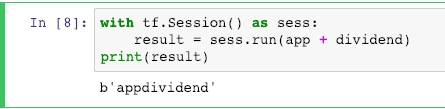Here, in the output, represents the byte literal.

Let’s perform the addition operation computation in Tensorflow. Write the following code in the next cell.

```a = tf.constant(10)
b = tf.constant(10)
with tf.Session() as sess:
result = sess.run(a + b)
print(result)```

Here, we have defined the two constants, which are integers, and then we have performed the addition operation inside the tensorflow session and stored the output in the result variable, and displayed that variable.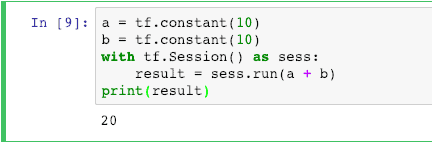## Create Matrix using Tensorflow

We can create a Matrix using Tensorflow functions like we have created using the NumPy library.

We can create the matrix using tf.fill() method provided by the tensorflow framework. See the following example.

```mat = tf.fill((5,5), 21)
with tf.Session() as sess:
op = sess.run(mat)
op```

See the output below.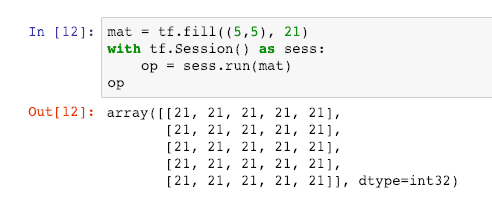In the above code, we have created the 5*5 matrix with the 21 values filled inside each element. Remember, we need to perform any operation inside the tensorflow sessions.

So these are some basics of Tensorflow.

That’s it.

This site uses Akismet to reduce spam. Learn how your comment data is processed.